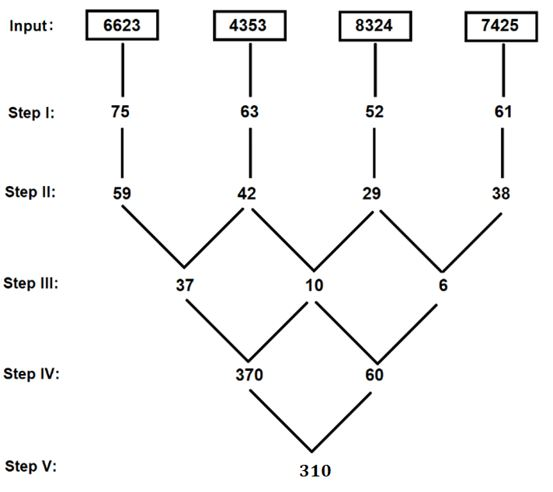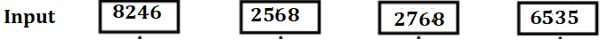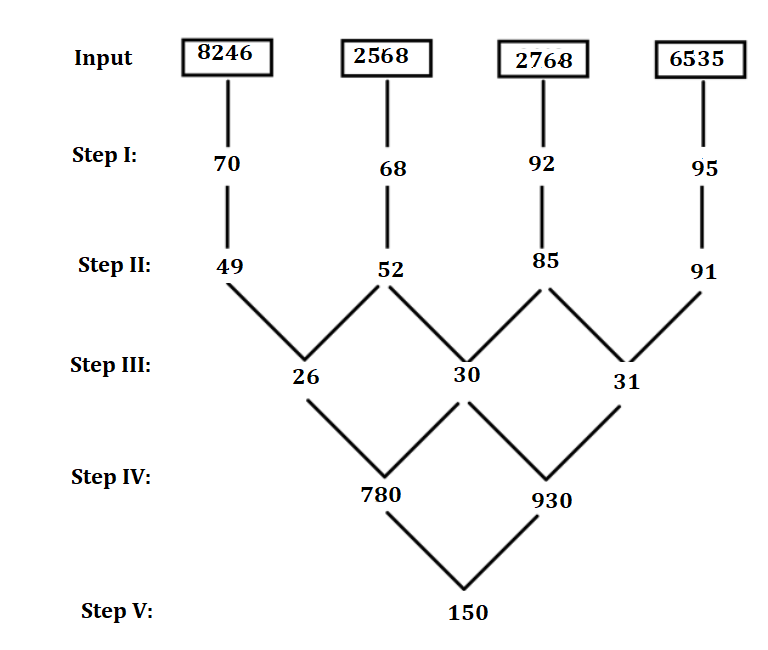Latest Banking jobs   »

# Reasoning Ability Quiz For SBI PO/Clerk Mains 2023- 6th January

Directions (1-5): When a word and number arrangement machine is given an input line of words and numbers, it arranges them following a certain rule. The following is an illustration of input and rearrangement. Read the following information and answer the questions that follow.

Input: grudge 38 rhapsody 42 71 bandit flakes 23 square 12
Step I: fquart 24 grudge 38 rhapsody 42 71 bandit flakes 23
Step 1: fquart 24 zhapsods 22 grudge 38 42 71 bandit flakes
Step III: fquart 24 zhapsods 22 frudgh 76 42 71 bandit flakes
Step IV: fquart 24 zhapsods 22 frudgh 76 tlakeg 84 71 bandit
Step V: fquart 24 zhapsods 22 frudgh 76 tlakeg 84 uandic 70

Step V is the last step of the output. Find the steps of the following input.

Input: 91 54 symphony cleanse block 67 mirror 88 48 painted

Q1. What is the last word in the last step of the given input?
(a) clock
(b) llock
(c) jlocc
(d) llocc
(e) llocd

Q2. What is the sum of the last three numbers in step IV?
(a) 333
(b) 401
(c) 332
(d) 244
(e) None of these

Q3. What is the 8th term from the right in step III?
(a) 108
(b) eainteq
(c) 53
(d) Cleanse
(e) None of these

Q4. Which of the following words does not appear in the last step?
(a) Zymphont
(b) Sirron
(c) Fleansed
(d) Eainteq
(e) None of the above

Q5. In which step does ‘sirron 66 91’ appear in the same order?
(a) Step I
(b) Step III
(c) Step IV
(d) Both step III and IV
(e) None of the above

Directions (6-10): The word and number arrangement machine when given an input line, rearranges them following a particular rule in each step. The following is an illustration of input and rearrangement.

Input: 89 47 taj sound 26 degree holding 27 fountain 52
Step I: fountain 52 89 47 taj sound 26 degree holding 27
Step II: fountain 52 holding 26 89 47 taj sound degree 27
Step III: fountain 52 holding 26 degree 27 89 47 taj sound
Step IV: fountain 52 holding 26 degree 27 taj 47 89 sound
Step V: fountain 52 holding 26 degree 27 taj 47 sound 89
Step VI: 14 52 08 26 05 27 20 47 19 89
Step VII: 10 14 24 24 10 18 06 22 30 34
Step VIII: 34 30 24 24 22 18 14 10 10 06

Step VIII is the last step of the above input. As per rules followed in the above steps, find out in each of the given questions the appropriate steps for the given input.

Input: junior 23 78 royalty result 55 72 expense 65 margin

Q6. Which of the following is the second last step of the given input?
(a) 10 10 18 18 18 30 10 22 06 30
(b) 10 10 18 30 18 18 10 22 06 30
(c) 30 30 22 18 18 18 10 10 10 06
(d) 30 30 18 18 10 22 18 10 10 06
(e) None of these

Q7. Which of the following element is 5th to the right of ‘royalty’ in step III of the given input?
(a) 55
(b) 65
(c) Expenses
(d) Result
(e) None of these

Q8. In which of the following step, ‘23 junior 72 78 royalty’ will appear in the same sequence?
(a) Step III
(b) Step IV
(c) Step II
(d) Step VI
(e) None of these

Q9. What is the sum of the numbers which are 4th from the both sides in step VI of the given arrangement?
(a) 87
(b) 80
(c) 73
(d) 77
(e) None of these

Q10. Which element comes exactly between the element which is third from the left and fourth from the right in the antepenultimate step?
(a) 18
(b) 72
(c) 55
(d) 23
(e) None of these

Directions (11-15): A arrangement of numbers is given as input. The further steps given are obtained by applying certain logic. Each step is resultant of previous step only.Note: 1st, 2nd, 3rd and 4th digits in any of the given step are taken from the left side of the given number. As per above applied logic in given illustration, find out the appropriate steps for the input given.Q11. Which of the following number is 2nd number from the left end in Step III?
(a) 37
(b) 26
(c) 30
(d) 31
(e) None of these

Q12. Which of the following number is 3rd number from the right end in Step II?
(a) 49
(b) 52
(c) 85
(d) 91
(e) None of these

Q13. What is the product of 2nd number from the left end in Step I and 1st number from the right end in Step III?
(a) 1256
(b) 2105
(c) 2108
(d) 2110
(e) None of these

Q14. Which of the following number is obtained in the final step?
(a) 150
(b) 140
(c) 160
(d) 120
(e) None of these

Q15. What is the difference between the number which is 4th from the right end in step I and 3rd from the left end in step II?
(a) 25
(b) 20
(c) 18
(d) 15
(e) None of these

Solutions

Solution (1-5):
Sol. The words are picked in the reverse alphabetical order. The first and the last letters of the word are exchanged and they are replaced with the letter that follows them alphabetically.
For example: symphony will be the first word picked in the given input. Then, the word will become yymphons and after the replacement y becomes z and s becomes t. i.e., the word becomes zymphont
The numbers are picked in the ascending order and placed immediately after the word picked. If it is an even number, it is doubled and if it is an odd number, then 1 is subtracted from it.

Input: 91 54 symphony cleanse block 67 mirror 88 48 painted
Step I: zymphont 96 91 54 cleanse block 67 mirror 88 painted
Step II: zymphont 96 eainteq 108 91 cleanse block 67 mirror 88
Step III: zymphont 96 eainteq 108 sirron 66 91 cleanse block 88
Step IV: zymphont 96 eainteq 108 sirron 66 fleansd 176 91 block
Step V: zymphont 96 eainteq 108 sirron 66 fleansd 176 llocc 90

S1. Ans. (d)
S2. Ans. (a)
S3. Ans. (b)
S4. Ans. (c)
S5. Ans. (b)

Solution (6-10):
For step I to V: The words are arranged in alphabetical order of 3rd last letter of the word.
Numbers are arranged from lowest to highest sum of digits. Both words and numbers are arranged alternatively.
For step VI: If number of letters in the words is even then the the alphabetic position of the last letter of the word (according to English alphabetical series) is written.
If number of letters in the word is odd then the alphabetic position of the first letter of the word (according to English alphabetical series) is written. The numbers are written as it is.
For Step VII: If the sum of digits of number is even then multiply the number by 3. If the sum of digits of number is odd then multiply the number by 2.
For Step VIII: Numbers are arranged in descending order from left to right.

Input: junior 23 78 royalty result 55 72 expense 65 margin
Step I: margin 23 junior 78 royalty result 55 72 expense 65
Step II: margin 23 junior 72 78 royalty result 55 expense 65
Step III: margin 23 junior 72 royalty 55 78 result expense 65
Step IV: margin 23 junior 72 royalty 55 expense 65 78 result
Step V: margin 23 junior 72 royalty 55 expense 65 result 78
Step VI: 14 23 18 72 18 55 05 65 20 78
Step VII: 10 10 18 18 18 30 10 22 06 30
Step VIII: 30 30 22 18 18 18 10 10 10 06

S6. Ans. (a)
S7. Ans. (b)
S8. Ans. (c)
S9. Ans. (d)
S10. Ans. (a)

Solution (11-15):
Step 1: First multiply the 1st, 2nd and 3rd digit of the given number and then add 4th digit to the resultant to obtain the number in Step I. For example, in the given input we have 5246 now, first multiply the 1st 2nd and 3rd digit of this number, so we get 5x2x4=40, then add the 4th digit of the given number to 40. So, finally we get 40 + 6 = 46.

Step II: In each number, square of 1st digit is added to double of 2nd digit to obtain the number in Step II. For example, for 46, square of 1st digit (4) is added to the double of 2nd digit = 16+12 = 28.
Step III: Difference between the product of 1st and 2nd digit of 1st number and product of 1st and 2nd digit of 2nd number (taking absolute value). The same operation is to be followed for 2nd and 3rd number and 3rd and 4th number.
Step IV: Product of 1st and 2nd number, and Product of 2nd and 3rd number to get the 1st and 2nd number in Step V.
Step V: Difference between 1st and 2nd number to obtain the final output.S11. Ans. (c)
S12. Ans. (b)
S13. Ans. (c)
S14. Ans. (a)
S15. Ans. (d)## FAQs

### Q1. What is the last word in the last step of the given input? (a) clock (b) llock (c) jlocc (d) llocc (e) llocd

S1. Ans. (d)

#### Congratulations!